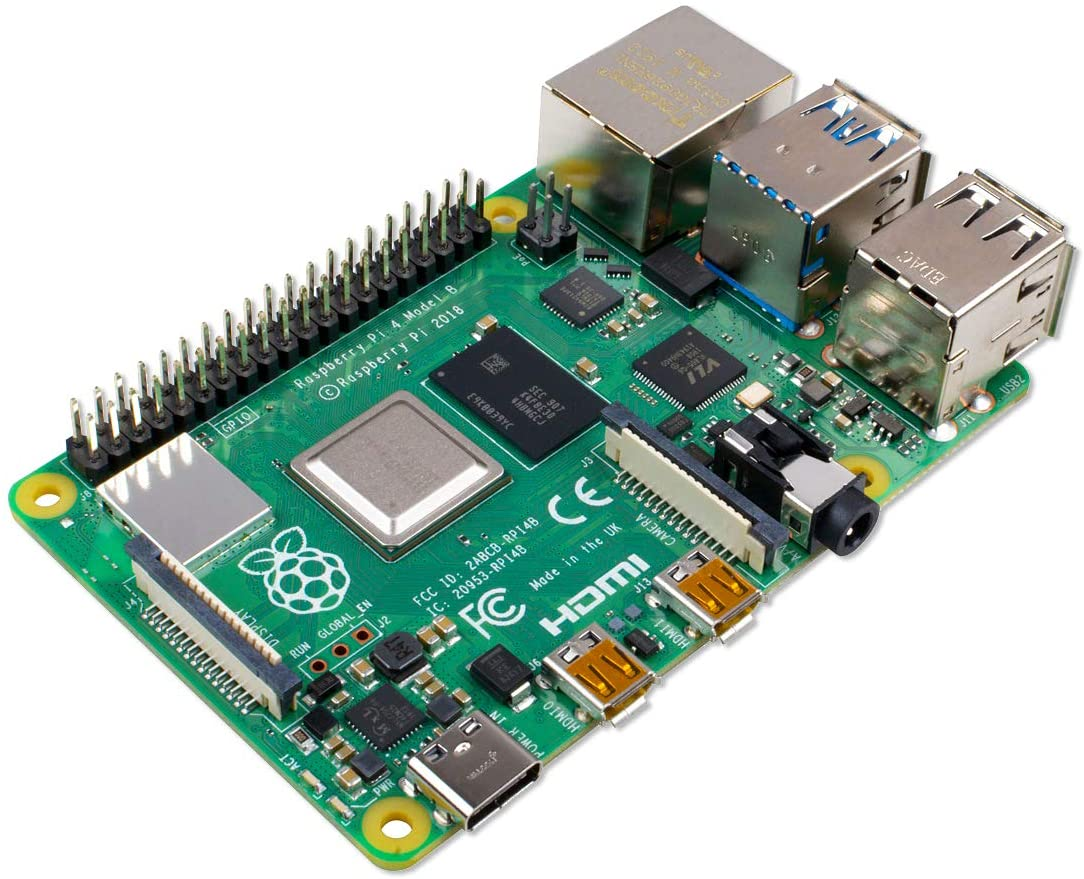# How to program a highly precise timer in C for Linux

I wanted to program a timer in C for my Raspberry Pi, which executes precise work instructions for 1 ms as well as for 0.1 ms. Unfortunately, since Python is compiled at runtime, Python was way too slow for this purpose. For this reason, the program was written in C. The 1 ms and 0.1 ms were confirmed after programming with the help of an oscilloscope.

In the following is an explanation why the library “sys/time.h” was used, as well as a code example with subsequent explanation. This code example is very well suited for bit-wise data transmission in communication technology.

This might also be interesting for you: How to program a highly precise Arduino Timer

## List of components

• Linux operating system (for example Raspberry Pi)
• Editor for C – Programming## The library „sys/time.h“

Functions like “usleep()” or “nanosleep()” would stop the complete program. For simple applications this might be sufficient, but for my purposes this was too imprecise. I wanted a timer that really works exactly in a 1 ms or 0.1 ms cycle. So instead of “usleep()” or “nanosleep()” another solution was chosen. The library “sys/time.h”. This library is able to read and compare the current “System Clock Time”.

## Code example for a precise timer in C

```#include <sys/time.h>

int main()
{
struct timeval tval_before, tval_after, tval_result;
int counter=0;
bool stop=false;

gettimeofday(&tval_before, NULL);
while(stop!=true)
{
gettimeofday(&tval_after, NULL);
timersub(&tval_after, &tval_before, &tval_result);
double time_elapsed = (double)tval_result.tv_sec + ((double)tval_result.tv_usec/1000000.0f);

while(time_elapsed < 0.001)  //1ms; you can change your desired time interval here
{
gettimeofday(&tval_after, NULL);
timersub(&tval_after, &tval_before, &tval_result);
time_elapsed = (double)tval_result.tv_sec + ((double)tval_result.tv_usec/1000000.0f);
}
gettimeofday(&tval_before, NULL);

if (counter==10000)
{
stop=true;
}

else
{
counter++;
}
}
return 0;
}
```

## Explanation of the code example:

A function “gettimeofday” writes the current system time into the variable “tval_before”. A While loop will then be executed until the actual task is completed.

Within the While loop the system time is stored again into a variable “tval_after”. Then the time difference between “tval_after” and “tval_before” is measured and stored in “tval_result”.

The next step of the timer in C is not immediately obvious: “tv_result” consists by definition of 2 parts. On the one hand a seconds part “.tv_sec” and on the other hand a microseconds part “.tv_usec”. This microsecond part has to be divided by one million to get the value in seconds. Afterwards, the microsecond part can be added to the second part.

The added value is called “time_elapsed”. If this value is less than one millisecond, another inner While loop is opened, which recalculates the value for “time_elapsed” until exactly 1 ms has passed. Afterwards, the value for “tval_before” is redefined using the “gettimeofday” function.

Since exactly 1 ms has passed at this point, the timer can now perform its actual operation. In this simple code example the variable “counter” is incremented by 1. That means for each interval step (in the code example 1 ms) the counter increases by 1. As soon as a fixed value for counter was reached the program stops. In this case the defined value is 10000. Then the While loop will be terminated. But this part of the code can be easily changed for your own purposes.

My measurements with an oscilloscope detected an exact frequency of 1 ms, even 0.1 ms was measured exactly. This code example is therefore also very suitable for an exact data transmission in the communication technology.

Cookie Consent with Real Cookie Banner